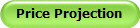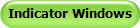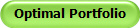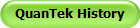# Omicron Research Institute

## QuanTek Features

QuanTek is a trading and portfolio management program based on the Science of Econometrics. It features a Price Projection that is derived from a Wavelet Adaptive Filter that we have developed. This filter is of the Least Mean Square (LMS) type and utilizes a wavelet decomposition of the prices and returns (over a 2048-day interval -- 8 years). This filter does a good job of finding the weak, time-dependent (non-stationary) correlations in the data, buried in the stochastic noise. The Price Projection from this filter provides an estimated return for each security, on a trading time scale from 1 to 128 days (10 days or greater recommended). The average absolute daily deviation of prices (volatility) is also measured, and these quantities are used to compute an Optimal Portfolio. Also, a set of Trading Rules is derived from a set of Technical Indicators that are obtained from the Wavelet Smoothing of the prices and returns (and also volatility).

QuanTek now works with either Alpha Vantage data (free) or EOD Historical Data (inexpensive), or directly with ASCII (CSV)files in a variety of formats. QuanTek can download Historical data, Daily Update data (actually going back 100 days), Intra-Day Update data, and also Fundamental data (not utilized at present). The Daily Update download can be scheduled at a fixed time every night, even if the computer is asleep (one security per minute). QuanTek can also export price data as a CSV file in its own special format, and then import this data again if needed. (This provides an easy way to save the downloaded data, or to correct the data if it is ever necessary.)

### Main Graph

One of the best features of QuanTek is the Main Graph. This graph continuously displays all 2048 days of data by means of a unique scrolling graph window that always keeps the graph centered on the screen. The prices displayed are corrected prices, corrected for splits and dividends. These corrected prices are the ones used for the Adaptive Filter and represent the actual historical value of the security. This graph shows the data on the same logarithmic scale(s) for all securities. Thus it is easy to compare different securities at a glance with regard to their historical return and volatility. This gives a good estimate of the future expected return and risk of each security compared to all the others.

Here is a more detailed description of the Main Graph:The Main Graph has five different scales, and enough ranges on each scale to cover the whole 2048-day data set. Each scale increases by a factor of two, and has different features displayed. One of the most important features of these graphs is that they all scale the same way on the horizongal and vertical axes, so the aspect ratio is preserved. That way, you can see at a glance the N-day return and volatility of each security relative to all other securities and all other graph scales. This emphasizes starkly how risky some securities are compared to others. A direct display of the risk is the width of the Bollinger Bands between different securities (on the same scale). The difference in risk between, say, an index and a penny stock is dramatic, but the usual types of displays do not often make this clear.

### Price Projection

The Price Projection is computed from a Wavelet Adaptive Filter of our own design, which is a Least Mean Square (LMS) type using regressors or Technical Indicators obtained by means of Wavelet Smoothing. The use of the wavelet decomposition results in a dramatic simplification of the filter design, and aids in separating the weak "signal" present in the data from the "noise". Basically, the wavelet decomposition separates the signal with respect to both frequency and time, with the frequency separation in octaves and the time separation longer on the low octaves and shorter on the high octaves. This is a natural separation for many real-world signals, as the only part of the signal that is actually relevant to the present time is that which occured most recently, on the order of the most recent few cycles on each octave. Then the Adaptive filter can utilize the most recent part of the signal on each octave level for regression. At present we use the wavelet decomposition of three different combinations of the price data, which we call the Relative Price (detrended prices), the Velocity (returns or 1st difference of prices) and Volatility (absolute deviation of prices). Use of the most recent values of the Relative Price, Velocity, and Volatility indicators as the regressors for the Wavelet Adaptive Filter results in a vast simplification of the filtering problem, as these three indicators are automatically orthogonal (uncorrelated) on each wavelet level. In addition, using the Volatility as one of the regressors makes the filter an implementation of GARCH (Generalized Auto-Regressive Conditional Heteroskedasticity).

Here is a more detailed description of the Price Projection:The Price Projection also displays Error Bars which represent the expected N-day future price range for N days in the future. This is based on the measured high-low range or Volatility of the past data, assumed to be scaled proportional to the square root of N. In addition, the actual N-day future price range for the past data is measured and may be displayed to compare with the estimated value. So this gives a direct indication of the expected future error bars of the Price Projection. The future projection may also be computed using several variants of the Wavelet Adaptive Filter, and the results of the different filters compared. In addition, the projections of these filters may be viewed at any point in the past, and then compared with the actual price action in the past.

### Indicator Windows

In addition to the Main Graph, the current version of QuanTek comes with a set of three Splitter Windows which are called the Indicator Windows. These three windows are called Indicators (low-pass smoothing), Indicators (band-pass smoothing), and Adaptive Filter Output. Each Indicator window has three splitter panes, displaying three separate indicators.

These Indicator Windows and their associated indicators are described in more detail here:The most important indicators are shown in the bottom panes of each window. These are the Relative Price (low-pass), Relative Price (band-pass), and Projected Future Returns. These three indicators are the source of the Buy/Sell Signals, Buy/Sell Points, and Long/Short Signals. The Indicator Windows are the source of the trading signals that are displayed on the Main Graph. They are also important diagnostic tools to check the output of the Adaptive Filter, as well as the regressors that are used in the filter calculation. The first two Indicator Windows display indicators built from the Relative Price, Velocity, and Volatility indicators, and these are precisely the regressors used in the Adaptive Filter calculation. The third window shows the actual output of the Adaptive Filter, along with N-day and 2048-day returns for comparison.

### Optimal Portfolio

One of the most important features of QuanTek is the Portfolio Optimization calculation. This is a standard calculation in Modern Portfolio Theory, typically used by mutual funds and other large money managers. This calculation is intended to design the portfolio so that the return is maximized while the risk is minimized. In order to do this, it is necessary to start with an estimated of the expected return and expected risk of each security in the portfolio. In QuanTek, the expected risk is taken to be the average absolute deviation of daily returns, which can be multiplied by the square root of N to get the expected N-day risk. The expected N-day return is what the Price Projection is designed to estimate. However, for the Portfolio Optimization calculation we use the average 2048-day return as the expected N-day return. This makes the Portfolio Optimization calculation independent of the Price Projection.

The Portfolio Optimization calculation is described in more detail here:The securities in the Optimal Portfolio are those in the Portfolio folder you happen to be in (excepting indexes). QuanTek also keeps track of the number of shares owned of each security, and the securities that have positions are a subset of the Optimal Portfolio called the Model Portfolio. You can then select those securities you wish to buy/sell and adjust the amounts of transactions to match the Optimal Portfolio. In this way you can arrive at a balanced portfolio.

The Optimal Portfolio may be viewed in the Portfolio Report, which is available from a toolbar button on the MainFrame toolbar. This is an *.rtf file that shows a variety of useful information, including the past returns on a variety of time scales, the N-day expected return from the Price Projection, and the standard deviation. Also shown is the Model Portfolio with the current price along with the basis price. Finally the Optimal Portfolio and Model Portfolio are shown together, along with the actual and optimal positions expressed as shares and percentage of the portfolio equity.

This information may also be viewed in the Short-Term Trades dialog, also available from a toolbar button. In addition, the Short-Term Trades dialog can be viewed anywhere in the program by pressing the Alt key. This dialog box is a handy quick reference for buy/sell decisions for the portfolio. In addition, there is a handy list box which estimates the expected average price and price range for the next day, which can be used for day trading. This next-day average price is estimated using the Standard LP filter.

## Statistical Tests

QuanTek has several Statistical Tests that are used mainly for checking that the Adaptive Filters and the Wavelet routines are working correctly. These are independent of the rest of the program and are not needed for trading  or investing decisions. But they give useful diagnostic information for test purposes.

### Correlation -- LP Filters/Indicators

The most important of these is the Correlation -- LP Filter test, which actually consists of two dialogs, the Indicators -- Linear Prediction Filters dialog and the Correlation Test -- LP Filters & Indicators dialog. The Indicators dialog displays the Adaptive Filter output (which needs to be computed first) or five other indicators, in the present or past, with various degrees of Wavelet smoothing (either low-pass or band-pass). After this is selected, the Correlation Test dialog creates a display of the correlation between the indicator and future returns, as a function of lead time, time horizon, correlation scale, and date in the historical past. The purpose of this dialog is to ferret out whatever real correlation exists between the indicators and future returns, as a function of time, and try to distinguish the real correlations from spurious ones. As stated, this test is mainly used to determine if the Adaptive Filter is actually effective at predicting future returns.

### Scatter Graph Indicators/Returns

Next, there is the Scatter Graph -- Returns dialog. This dialog measures the correlation directly between two securities, or the same security, in which you can vary the time lag between the two. The result is displayed in the form of a scatter graph, with a point on the graph for each point of the data. If there is correlation, it can be seen as a non-uniform distribution of the points on the graph. The actual degree of correlation is displayed, computed three different ways, along with the confidence level that the correlation is not spurious. There is also another dialog connected with this one, that displays the auto-correlation or cross-correlation sequence between the same two securities, as a function of time lag. This correlation uses the Fourier transform method. The Scatter Graph -- Returns dialog could be useful for choosing securities in the portfolio to achieve maximum diversification, since to achieve this it is important to find pairs of securities that are anti-correlated.

### Wavelet Analysis/Variance

Two other statistical tests are the Wavelet Analysis and Wavelet Variance dialogs. These are mainly used to test whether the Wavelet routines are functioning correctly, and to display their output graphically. The Wavelet Analysis dialog displays the output of the Wavelet filters in terms of the wavelet coefficients and the multi-resolution analysis (MRA). The Wavelet Variance dialog uses the actual price data to compute its wavelet variance and then display a covariance matrix based upon it.

### Periodogram/Wavelet Spectrum

Finallythere are two statistical tests that show a standard spectral decomposition of the price returns. The Periodogram Spectrum displays a standard Fourier spectrum of the returns. This is a standard computation in time-series analysis. The Wavelet Spectrum displays the corresponding Wavelet spectrum of the returns, averaged over time, so there is one average value per frequency octave. Theoretically, if the returns data are correlated (assumed stationary, so the correlation is time-independent), then the correlation would show up in a non-constant spectrum of the returns. On the other hand, if the spectrum is constant, then the returns are "white noise", in accordance with the Random Walk model. Actually, though, the theoretical variance of the spectrum is about 100%, so it is hard to tell from the spectral graph alone whether there are really any meaningful correlations in the data. (Also, the correlation is not expected to be stationary.) This is why the Correlation test described above is more relevant for non-stationary correlations.

## QuanTek History

Here is a summary of the history of the QuanTek program, going back to the late-90s:The QuanTek program started in the mid-90s as a simple Technical Analysis program and programming exercise. In incorporated a "canned" Linear Prediction filter which I now call the Standard LP filter. This is actually the Burg Gradient-Adaptive Lattice (GAL) filter. This is a nicely designed filter which works well, but I came to the conclusion that it was not well adapted to noisy financial data. It seems to perform best on smooth data with a high signal to noise ratio (S/N). So a decided to design my own filter which would be better adapted to finding weak correlations in noisy financial data. The use of Wavelet techniques seemed attractive for ferreting out the weak signal from the noise. Then I used a standard Least-Mean Square (LMS) filter with regressors obtained using Wavelet smoothing of the data in various ways. The result is a stable filter that seems to perform well. The rest of the program basically serves to download and prepare the data for use in the filter, and display the data and Price Projection in a variety of graphs.

The other aspect of QuanTek is its Optimal Portfolio calculation. This has evolved over time as well. Finally we had to derive our own method from the standard Markowitz method, which is explained in the article on Portfolio Optimization (under Articles and in the Help file). So the QuanTek program has evolved over time and improved as our knowledge of the various subject areas has improved, such as Econometrics and Adaptive Filters. I think this program has now reached the state-of-the-art and I intend to continue to improve QuanTek so as to keep it on the cutting edge!